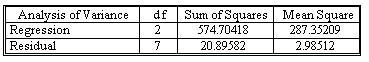### Why should I choose AnalystNotes?

AnalystNotes specializes in helping candidates pass. Period.

##### Subject 4. The Standard Error of Estimate in Multiple Linear Regression Model
The least-squares estimates of the population parameters β0, β1, ..., βk are the values b0, b1, ..., bk that minimize the residual sum of squares SSE, where

SSE = ∑(yt - y-hatt)2 = ∑(yt - b0 - b1x1t - b2x2t - ... - bkxkt)2

If we estimate the multiple regression model by the method of least squares and if the model contains a constant term, then we obtain

∑e-hatt = 0

that is, the sum of the residuals is always 0 provided the sample regression model contains a constant term b0.

To test hypotheses and to construct confidence intervals, we need an estimate of σe2. Recall that in the simple leaner regression model an unbiased estimator of σe2 is se2 = SSE/(t-2), where t - 2 is the degrees of freedom of the model. An unbiased estimator of σe2 is defined in an analogous manner in the multiple regression model. In the formula for se2, the denominator is t minus the number of coefficients that have to be estimated. In the multiple regression model, there are (k + 1) coefficients β0, β1, ..., βk to be estimated, and so the denominator in the formula for se2 is t - (k + 1).

The standard error of estimate (SEE) of the regression is:The value t - k - 1 is the degrees of the freedom of the regression equation, in a sense we use up (k + 1) degrees of freedom before calculating SSE because we first estimate the (k + 1) coefficients β0, β1, ..., βk.

Let's continue with the example in los a.

The ANOVA output reports quantities related to the overall explanatory power of the regression.An unbiased estimate of the variance of the errors is se2 = SSE /(t - k - 1) = 20.8958/(10 - 3) = 2.9851, and the standard error of estimate is se = (2.9851)1/2 = 1.7277.

Based on the empirical rule, for a normal distribution, approximately 95% of the error terms should be less than 2se (or 3.4554) units from the estimated plane and the approximately 95% of the sample observations should lie within 3.4554 units of the estimated plane.

Learning Outcome Statements

e. calculate and interpret a predicted value for the dependent variable, given an estimated regression model and assumed values for the independent variables;

CFA® 2023 Level I Curriculum, Volume 1, Module 2

User Comment
katybo t-k-1 = residual degrees of freedom